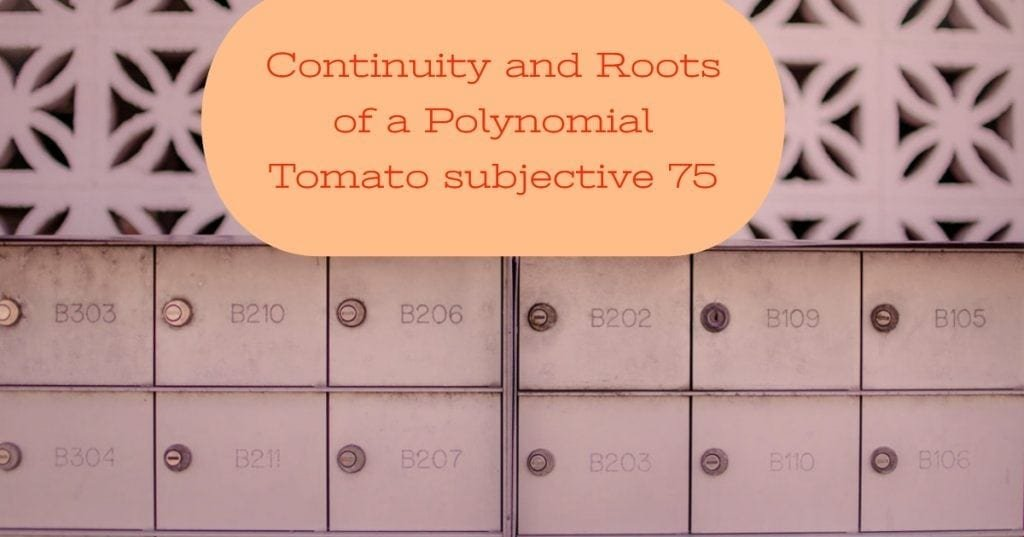How Cheenta works to ensure student success?
Explore the Back-Story

# Test of Mathematics Solution Subjective 75This is a Test of Mathematics Solution Subjective 75 (from ISI Entrance). The book, Test of Mathematics at 10+2 Level is Published by East West Press. This problem book is indispensable for the preparation of I.S.I. B.Stat and B.Math Entrance.

Also visit: I.S.I. & C.M.I. Entrance Course of Cheenta

## Problem

Show that there is at least one value of ${x}$ for which ${\displaystyle{\sqrt{x}} + {\sqrt{x}} = 1}$

## Solution

${\displaystyle{\sqrt{x}} + {\sqrt{x}} = 1}$
${\displaystyle{\sqrt{x}} + {\sqrt{x}} - 1}$ is a continuous function. Now if we can chose ${\displaystyle{\sqrt{x}} + {\sqrt{x}} - 1}$ takes both negative & positive numbers then the ${\displaystyle{\sqrt{x}} + {\sqrt{x}} - 1 = 0}$ have a solution.

For ${\displaystyle{x = {\frac{1}{64}}}}$, ${\displaystyle{\sqrt{x}} + {\sqrt{x}} - 1 < 0}$ & for ${x = 64}$, ${\displaystyle{\sqrt{x}} + {\sqrt{x}} - 1 > 0}$.
So by intermediate value theorem we can say that ${\displaystyle{\sqrt{x}} + {\sqrt{x}} - 1 = 0}$ has a solution for some real ${x}$.

## Important Resources:This is a Test of Mathematics Solution Subjective 75 (from ISI Entrance). The book, Test of Mathematics at 10+2 Level is Published by East West Press. This problem book is indispensable for the preparation of I.S.I. B.Stat and B.Math Entrance.

Also visit: I.S.I. & C.M.I. Entrance Course of Cheenta

## Problem

Show that there is at least one value of ${x}$ for which ${\displaystyle{\sqrt{x}} + {\sqrt{x}} = 1}$

## Solution

${\displaystyle{\sqrt{x}} + {\sqrt{x}} = 1}$
${\displaystyle{\sqrt{x}} + {\sqrt{x}} - 1}$ is a continuous function. Now if we can chose ${\displaystyle{\sqrt{x}} + {\sqrt{x}} - 1}$ takes both negative & positive numbers then the ${\displaystyle{\sqrt{x}} + {\sqrt{x}} - 1 = 0}$ have a solution.

For ${\displaystyle{x = {\frac{1}{64}}}}$, ${\displaystyle{\sqrt{x}} + {\sqrt{x}} - 1 < 0}$ & for ${x = 64}$, ${\displaystyle{\sqrt{x}} + {\sqrt{x}} - 1 > 0}$.
So by intermediate value theorem we can say that ${\displaystyle{\sqrt{x}} + {\sqrt{x}} - 1 = 0}$ has a solution for some real ${x}$.

## Important Resources:

### Knowledge Partner